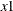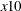### BOUNDS Statement

`BOUNDS` bound1 <, bound2 …> ;

The BOUNDS statement imposes simple boundary constraints on the parameter estimates. BOUNDS statement constraints refer to the parameters estimated by the ENTROPY procedure. You can specify any number of BOUNDS statements.

Each boundary constraint is composed of variables, constants, and inequality operators in the following form:

`item operator item <,operator item <,operator item …> >`

Each item is a constant, the name of a regressor variable, or a list of regressor names. Each operator is <, >, <=, or >=.

You can use either the BOUNDS statement or the RESTRICT statement to impose boundary constraints; the BOUNDS statement provides a simpler syntax for specifying inequality constraints. See section RESTRICT Statement for more information about the computational details of estimation with inequality restrictions.

Lagrange multipliers are reported for all the active boundary constraints. In the printed output and in the OUTEST= data set, the Lagrange multiplier estimates are identified with the names BOUND1, BOUND2, and so forth. The probability of the Lagrange multipliers are computed using a beta distribution (LaMotte, 1994). Nonactive or nonbinding bounds have no effect on the estimation results and are not noted in the output. To give the constraints more descriptive names, use the RESTRICT statement instead of the BOUNDS statement.

The following BOUNDS statement constrains the estimates of the coefficients of WAGE and TARGET and the 10 coefficients ofthroughto be between zero and one. This example illustrates the use of parameter lists to specify boundary constraints.

```   bounds 0 < wage target x1-x10 < 1;
```

The following is an example of the use of the BOUNDS statement to impose boundary constraints on the variables `X1`, `X2`, and `X3`:

```proc entropy data=zero;
bounds .1 <= x1 <= 100,
0 <= x2 <=  25.6,
0 <= x3 <=   5;

model y = x1 x2 x3;
run;
```

The parameter estimates from this run are shown in Figure 13.23.

Figure 13.23: Output from Bounded Estimation

 Prior Distribution of Parameter T

The ENTROPY Procedure

Variables(Supports(Weights)) x1 x2 x3 Intercept y

 Prior Distribution of Parameter T

The ENTROPY Procedure
GME Estimation Summary

Data Set Options
DATA= WORK.ZERO

Minimization Summary
Parameters Estimated 4
Covariance Estimator GME
Entropy Type Shannon
Entropy Form Dual
Numerical Optimizer Newton-Raphson

Final Information Measures
Objective Function Value 6.292861
Signal Entropy 6.375715
Noise Entropy -0.08285
Normed Entropy (Signal) 0.990364
Normed Entropy (Noise) 1.004172
Parameter Information Index 0.009636
Error Information Index -0.00417

Observations
Processed
Used 20

 NOTE: At GME Iteration 20 convergence criteria met.

GME Summary of Residual Errors
Equation DF Model DF Error SSE MSE Root MSE R-Square Adj RSq
y 4 16 1665620 83281.0 288.6 -0.0013 -0.1891

GME Variable Estimates
Variable Estimate Approx Std Err t Value Approx
Pr > |t|
Label
x1 0.1 0 . .
x2 0 0 . .
x3 3.33E-16 0 . .
Intercept -0.00432 3.406E-6 -1269.3 <.0001
1.25731 9130.3 0.00 0.9999 0.1 <= x1
0.009384 0 . . 0 <= x2
0.000025 0 . . 0 <= x3